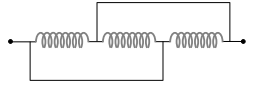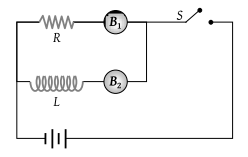Two coils of self inductance L1 and L2 are placed closer to each other so that total flux in one coil is completely linked with other. If M is mutual inductance between them, then

(1) M = L1 L2

(2) M = L1/L2

(3) $M=\sqrt{{L}_{1}{L}_{2}}$

(4) M = (L1 L2)2

Concept Questions :-

Mutual-inductance
High Yielding Test Series + Question Bank - NEET 2020

Difficulty Level:

Two circuits have coefficient of mutual induction of 0.09 henry. Average e.m.f. induced in the secondary by a change of current from 0 to 20 ampere in 0.006 second in the primary will be

(1) 120 V

(2) 80 V

(3) 200 V

(4) 300 V

Concept Questions :-

Mutual-inductance
High Yielding Test Series + Question Bank - NEET 2020

Difficulty Level:

A coil and a bulb are connected in series with a dc source, a soft iron core is then inserted in the coil. Then

(1) Intensity of the bulb remains the same

(2) Intensity of the bulb decreases

(3) Intensity of the bulb increases

(4) The bulb ceases to glow

Concept Questions :-

Faraday 's law and lenz law
High Yielding Test Series + Question Bank - NEET 2020

Difficulty Level:

The inductance of a coil is 60μH. A current in this coil increases from 1.0 A to 1.5 A in 0.1 second. The magnitude of the induced e.m.f. is

(1) 60 × 10–6 V

(2) 300 × 10–4 V

(3) 30 × 10–4 V

(4) 3 × 10–4 V

Concept Questions :-

Faraday 's law and lenz law
High Yielding Test Series + Question Bank - NEET 2020

Difficulty Level:

The self inductance of a coil is L. Keeping the length and area same, the number of turns in the coil is increased to four times. The self inductance of the coil will now be

(1) $\frac{1}{4}L$

(2) L

(3) 4 L

(4) 16 L

Concept Questions :-

Self-inductance
High Yielding Test Series + Question Bank - NEET 2020

Difficulty Level:

A coil has an inductance of 2.5 H and a resistance of 0.5 r. If the coil is suddenly connected across a 6.0 volt battery, then the time required for the current to rise 0.63 of its final value is

(1) 3.5 sec

(2) 4.0 sec

(3) 4.5 sec

(4) 5.0 sec

Concept Questions :-

LR circuit
High Yielding Test Series + Question Bank - NEET 2020

Difficulty Level:

Pure inductance of 3.0 H is connected as shown below. The equivalent inductance of the circuit is(1) 1 H

(2) 2 H

(3) 3 H

(4) 9 H

Concept Questions :-

Self-inductance
High Yielding Test Series + Question Bank - NEET 2020

Difficulty Level:

If a current of 10 A flows in one second through a coil, and the induced e.m.f. is 10 V, then the self-inductance of the coil is

(1) $\frac{2}{5}H$

(2) $\frac{4}{5}H$

(3) $\frac{5}{4}H$

(4) 1 H

Concept Questions :-

Self-inductance
High Yielding Test Series + Question Bank - NEET 2020

Difficulty Level:

The adjoining figure shows two bulbs B1 and B2 resistor R and an inductor L. When the switch S is turned off(1) Both B1 and B2 die out promptly

(2) Both B1 and B2 die out with some delay

(3) B1 dies out promptly but B2 with some delay

(4) B2 dies out promptly but B1 with some delay

Concept Questions :-

Self-inductance
High Yielding Test Series + Question Bank - NEET 2020

Difficulty Level:

An inductance L and a resistance R are first connected to a battery. After some time the battery is disconnected but L and R remain connected in a closed circuit. Then the current reduces to 37% of its initial value in

(1) RL sec

(2) $\frac{R}{L}sec$

(3) $\frac{L}{R}sec$

(4) $\frac{1}{LR}sec$

Concept Questions :-

LR circuit